Smartick is an advanced online program that teaches kids math and coding in only 15 min. a day
Try it for free!

# I want to learn about: Learning Resources

Teaching resources for elementary mathematics from Smartick. Here you will find posts about teaching resources to make learning mathematics easier and more fun.

May18

## Learn Everything About the Properties of Powers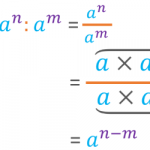In this post, we are going to explain the properties of powers. We will also try to justify the reasoning for following them. Before we begin, let’s remind ourselves of what a power is. What Is a Power? A power is the product of a number multiplied by itself a certain number of times. In […]

Continue reading »

May04

## Incomplete Horizontal Addition and Subtraction to Develop Mathematical Flexibility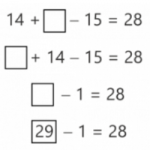Today we are going to solve incomplete horizontal addition and subtraction exercises by using different strategies. In mathematics, it is important to know how to use different strategies for solving the same problem or exercise. Knowing various strategies, how to apply them, and knowing when is the best time to use each, is what we […]

Continue reading »

Apr20

## Adding Fractions with Different Denominators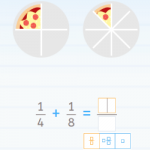We are going to begin this post about adding fractions with three very simple questions: 1. If you had one apple and were given two apples, how many apples do you have now? 2. If you had one apple and were given half an apple, how many apples do you have now? 3. If you […]

Continue reading »

Apr13

## Elementary Geometry – Open LinesIn this post, we are going to discover what an open line is and how it is different from a closed line. We will also see different types of open lines. Before we begin, take a look at this post about straight and curved lines to refresh your memory. Open Lines We know that a […]

Continue reading »

Apr06

## The Reason for Divisibility Criteria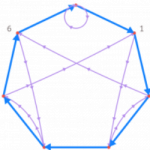Sometimes we may be interested in knowing if one number is divisible by another, without having to perform a division. This is especially useful when we work with large numbers that require a lot of time for completing calculations. If you would like to refresh your memory on divisibility criteria, take a look at this […]

Continue reading »

Mar23

## Identifying Flat Symmetrical FiguresIn today’s post, I am going to present a series of exercises to practice how to identify flat symmetrical figures. To begin, I recommend that if you don’t remember symmetry or the characteristics that make a figure symmetrical very well, take a look at this previous post. You’ll be able to review all of the […]

Continue reading »

Mar16

## Equivalent Fractions on a Number Line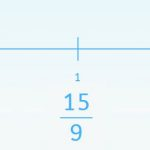Today we are going to learn how to solve activities related to the representation of fractions on a number line and the amplification and simplification of fractions. To do this we need to use equivalent fractions. It is easy to represent a fraction on a number line when it is divided into the same number […]

Continue reading »

Mar09

## Reducible Equations: What Are They? How Do We Solve Them?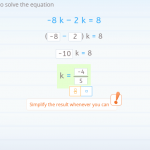In this post, we are going to explain reducible equations with several examples taken from Smartick’s daily sessions. We will begin with some easier examples, and work our way toward those that are more complicated. If you need to review what an equation is, and its terms, you can take a look at this previous […]

Continue reading »

Feb17

## Working with Venn Diagrams in SmartickIn earlier posts, we have learned about set theory and Venn diagrams. Today, we are going to look at some examples of classification using Venn diagrams in Smartick. Attributes The method that we use to understand whether or not the elements that we want to classify meet some characteristics are what we call attributes. The […]

Continue reading »

Feb03

## Working on Combined Operations through Word ProblemsIn today’s post, we are going to present the way that we work on combined operations through word problems in Smartick. In the field of mathematics in school, combined operations are those problems where we need to use more than one operation. If you don’t remember exactly what they were, click here to refresh your memory! […]

Continue reading »

Jan06

## How to Calculate a Perimeter: Part 2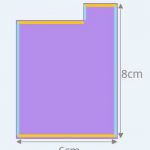In an earlier post, we learned how to calculate the perimeter of any polygon and formulas for regular polygons and rectangles. Today, we are going to learn how to calculate the perimeter of other types of polygons: rhombuses, isosceles triangles, isosceles trapezoids, and stepped polygons. NOTE TO OUR READERS IN THE U.K. – This post […]

Continue reading »

Dec23

## Learning Math in the Early Years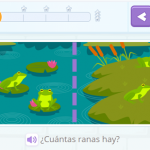When thinking about math in the early years, math for little ones, we contemplate counting, first numbers, and acquiring numerical sequence. And indeed, it is at this stage where we begin to develop the ability to count. But these are not the only math topics that children can learn in the early years. There is […]

Continue reading »